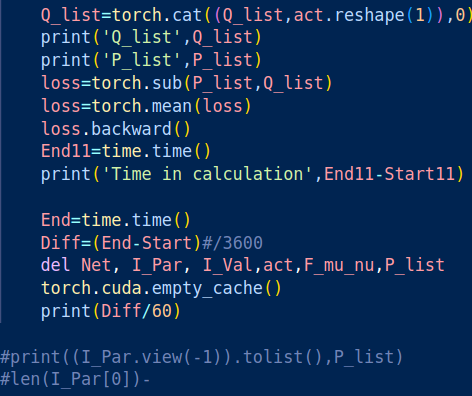# Custom Loss in autoregressive neural network

Hi, I am working with Auto regressive neural network, which is based on conditional probability. I have a 1d tensor which consist of [1,-1] and total 2500 elements in it like[1,-1,1,1,-1,…]. This is the input and output of my network is between [0,1] of same 2500 elements.
Now I take the first element of my output and determine the first element of my input using random sampling (say my first element of output is 0.5365 then I create a random number between 0 and 1 if this random number is less then 0.5365 then first element of input is -1 and if it is greater, then it is 1).
I do this 2500 time. In the last iteration, i got the final sets of input and output. I use this to determine the loss. My loss is:
Loss=sum of final inputs - log(sum of final outputs). Now, what do next, like how to calculate the gradient, loss function, back propagation etc. and all the other operations.

Could you post your current code (or a pseudo code) showing how exactly you are calculating the model output and loss?
Generally, you should be able to directly call `backward()` on the output tensor assuming you’ve used differentiable PyTorch operations in the forward pass and didn’t detach the computation graph explicitly.

Hi, ptrblck

this is my class of neural network,n and m controls the size of the input tensor nmmmm is 2500

in this I am doing my calculation for conditional probability. In the 2nd line I created instance for the Neural Network as Net. P_list is a tensor for all the probabilities stored that I will need in calculation for loss.In this, Q_list is a tensor which contains the final configuration [1,-1,1,1,1,-1,…].
I am doing the calculations on GPU in batches that’s why I am taking mean.
After applying loss.backword(). I am getting a runtime error:

UserWarning: Error detected in AddmmBackward0. Traceback of forward call that caused the error:
File “/hac/home/vchahar/gauge_neural.py”, line 58, in
Net_1=(Net(I_Par))#forward pass of the neural network
File “/hac/home/vchahar/.local/lib/python3.10/site-packages/torch/nn/modules/module.py”, line 1501, in _call_impl
return forward_call(*args, **kwargs)
File “/hac/home/vchahar/py_func.py”, line 37, in forward
y=self.fc1(x)
File “/hac/home/vchahar/.local/lib/python3.10/site-packages/torch/nn/modules/module.py”, line 1501, in _call_impl
return forward_call(*args, **kwargs)
File “/hac/home/vchahar/.local/lib/python3.10/site-packages/torch/nn/modules/linear.py”, line 114, in forward
return F.linear(input, self.weight, self.bias)
Variable._execution_engine.run_backward( # Calls into the C++ engine to run the backward pass
Traceback (most recent call last):
File “/hac/home/vchahar/gauge_neural.py”, line 109, in
loss.backward(retain_graph=True)
File “/hac/home/vchahar/.local/lib/python3.10/site-packages/torch/_tensor.py”, line 487, in backward
File “/hac/home/vchahar/.local/lib/python3.10/site-packages/torch/autograd/init.py”, line 200, in backward
Variable._execution_engine.run_backward( # Calls into the C++ engine to run the backward pass
RuntimeError: one of the variables needed for gradient computation has been modified by an inplace operation: [torch.cuda.FloatTensor [4, 2500]] is at version 5000; expected version 4998 instead. Hint: the backtrace further above shows the operation that failed to compute its gradient. The variable in question was changed in there or anywhere later. Good luck!

Could you post the code by wrapping it into three backticks ``` instead of screenshots as it would be easier to debug it?

Sure,

def init(self,n,m):
self.n=n
self.m=m
self.in_size=nmmmm

``````    self.fc1=nn.Linear(self.in_size,self.in_size,bias=False)
nn.init.xavier_uniform_(self.fc1.weight)

self.fc2=nn.Linear(self.in_size,self.in_size,bias=False)
nn.init.xavier_uniform_(self.fc2.weight)

self.out=nn.Linear(self.in_size,self.in_size,bias=False)
nn.init.xavier_uniform_(self.out.weight)

self.prelu=nn.PReLU()
self.sig=nn.Sigmoid()

def forward(self,x):
y=self.fc1(x)
z=self.prelu(y)
y1=self.fc2(z)
z1=self.prelu(y1)
x1=self.out(z1)
x2=self.sig(x1)
return x2 ""
``````

This is Neural Network class

"Start1=time.time()
I_Par=torch.zeros((j,mmmmn)).to(dev)#rand_mat(j,m,n,I_Val).to(dev)
print(I_Par.size())
P_list=torch.empty([len(I_Par),j]).to(dev)#torch.as_tensor([]).to(dev)
print(P_list.size())
Endi=time.time()

``````print('Time in initialize a tensor and transferring to gpus ',Endi-Start1)

Start2=time.time()
for i in range(len(I_Par)):
print(i)
rand=torch.rand(j).to(dev)

#create the instance of beural network class
Net_1=(Net(I_Par))#forward pass of the neural network

comparison=torch.gt(Net_1[:,i],rand)

I_Par[:,i][comparison]=0
I_Par[:,i][~comparison]=2

P_list[i,:]=Net_1[:,i]
End2=time.time()
print('Time in network',End2-Start2)

print(I_Par)
P_list1=torch.log(P_list)
P_list2=torch.sum(P_list1,dim=0)
Q_list=torch.as_tensor([]).to(dev) "
``````

and the last part is

“Q_list=torch.cat((Q_list,act.reshape(1)),0)
print(‘Q_list’,Q_list)
print(‘P_list2’,P_list2)
loss=P_list2-Q_list
loss=torch.mean(loss)
loss.backward()
optimizer.step()
End11=time.time()
print(‘Time in calculation’,End11-Start11)”

the error showing is

/hac/home/vchahar/.local/lib/python3.10/site-packages/torch/autograd/init.py:200: UserWarning: Error detected in MmBackward0. Traceback of forward call that caused the error:
File “/hac/home/vchahar/gauge_neural.py”, line 59, in
Net_1=(Net(I_Par))#forward pass of the neural network
File “/hac/home/vchahar/.local/lib/python3.10/site-packages/torch/nn/modules/module.py”, line 1501, in _call_impl
return forward_call(*args, **kwargs)
File “/hac/home/vchahar/py_func.py”, line 37, in forward
y=self.fc1(x)
File “/hac/home/vchahar/.local/lib/python3.10/site-packages/torch/nn/modules/module.py”, line 1501, in _call_impl
return forward_call(*args, **kwargs)
File “/hac/home/vchahar/.local/lib/python3.10/site-packages/torch/nn/modules/linear.py”, line 114, in forward
return F.linear(input, self.weight, self.bias)
Variable._execution_engine.run_backward( # Calls into the C++ engine to run the backward pass
Traceback (most recent call last):
File “/hac/home/vchahar/gauge_neural.py”, line 110, in
loss.backward()
File “/hac/home/vchahar/.local/lib/python3.10/site-packages/torch/_tensor.py”, line 487, in backward